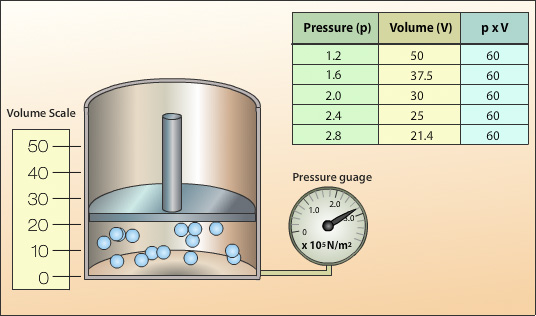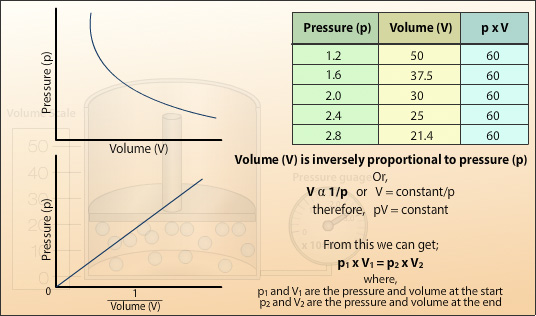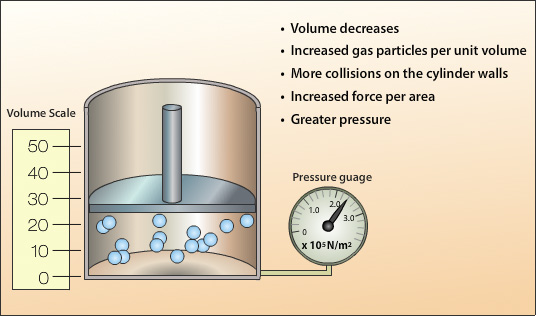## Boyle's law

All the particles (atoms and molecules) of a substance are continually moving and so possess kinetic energy. In gases the movement of the particles is highly energetic and this is the reason why gases form, the particles have enough energy to overcome the attractive forces holding the particles together. In gases the particles are moving very quickly and freely in a random manner constantly bumping into each other and their surroundings. It is these collisions between the particles of the gas and the walls of the container it is confined to that creates gas pressure. The gas pressure is the overall force of all these collisions divided by the area of the walls of the container it is confined in.

The relationship of a gas with pressure and volume was developed by the scientist Robert Boyle at around 1660 and is known as Boyle’s Law.

Boyle’s law states:

"For a fixed mass of gas, at a constant temperature, the product (pressure x volume) is a constant."

Pressure x Volume = constant

p x V = constant

The animation below gives and explanation of Boyle's law:A sealed cylinder with no leaks contains a fixed mass of a gas kept at a constant temperature. The gas pressure is created by the collision of the moving gas particles with each other and against the walls of the cylinder.The above set up is used to investigate the relationship between pressure and volume for a gas. A force is exerted on the piston to compress the gas. The corresponding pressure and volume values are recorded for different applied forces.By plotting the recorded values of pressure (p) against volume (V) a curve is produced. We can see from the values that when the pressure is doubled the volume is halved. If the pressure was to increase by 3 the volume would decrease to a third. Thus, the volume is inversely proportional to the pressure. By plotting pressure (p) against the reciprocal of the volume (1/V) a straight line is obtained the gradient of which is the constant in Boyle’s Law.A decrease in volume increases the number of gas particles per unit volume. This results in an increase in the number of gas particles close to the cylinder walls and therefore an increase in the number of collisions with the wall. As the number of collisions per unit area increases so does the force per unit area thereby giving an increase in pressure.

## Boyle’s Law Example:

Using the example of the sealed cylinder above, the volume of gas at the start is 50 cm3 with a pressure of 1.2 x 105 Pascals. The piston is pushed slowly into the syringe until the pressure on the gauge reads 2.0 x 105 Pascals. What is the volume of gas?

### Solution:

We know

p x V = constant

therefore,

p1 x V1 = p2 x V2

p1= 1.2 x 105 Pascals
V1 = 50 cm3
p2 = 2.0 x 105 Pascals
V2 =
?

p1 x V1 = p2 x V2

 V2 = p1x V1 p2

 V2 = 1.2 x 105 x 50 2.0 x 105

V2 = 30 cm3